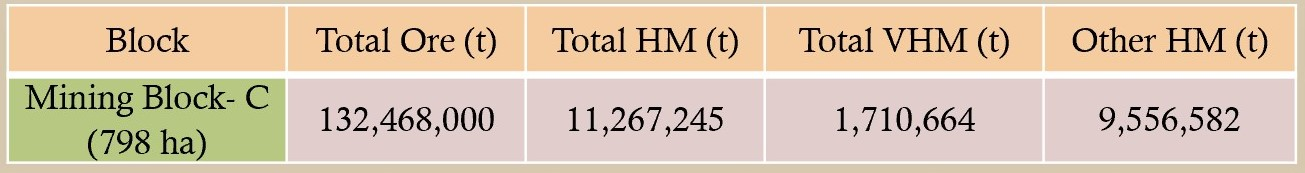• The Average Density of Sand is 1.66 ton/M3
• Method of Section was applied to calculated mineral resource estimation
• The 3 Proposed Mining Blocks fall within a very active and gigantic river system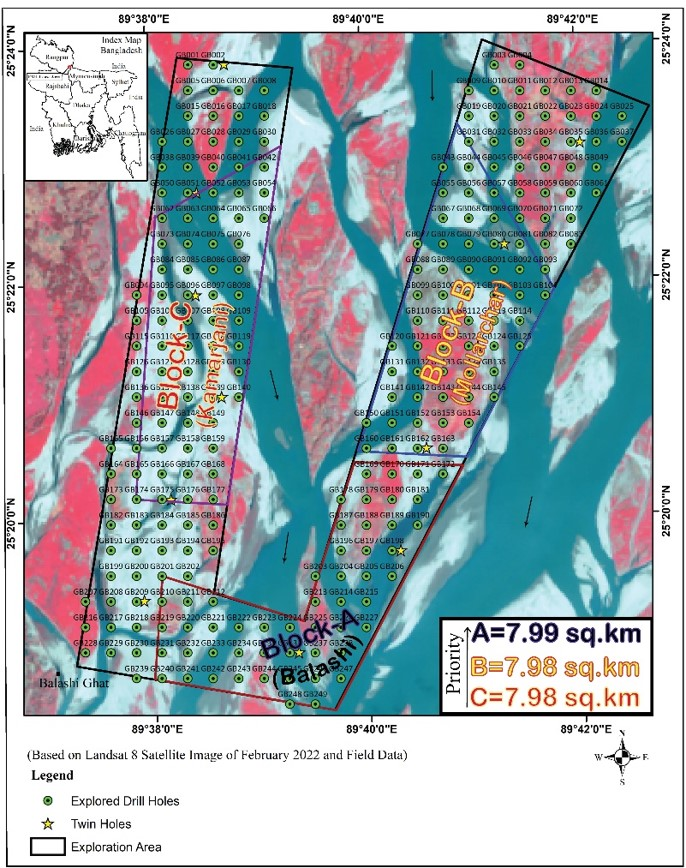• The river is carrying about 0.8 billion tonne of sediment each year
• Resource for the proposed Mining Block-A up to a depth of 10 meters have been calculated using 8.15% weighted average of HM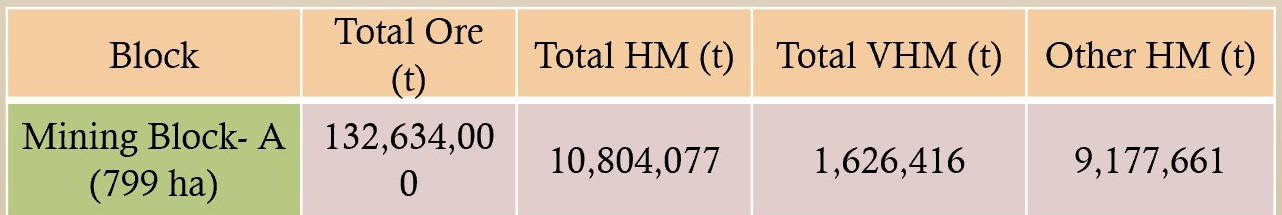• Resource for the proposed Mining Block-B up to a depth of 10 meters have been calculated using 8.05% weighted average of HM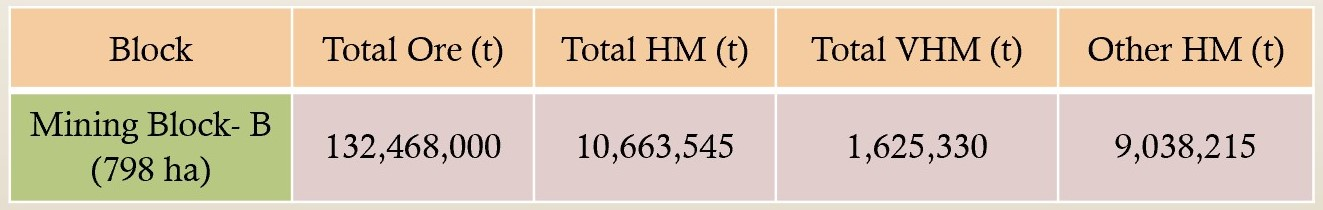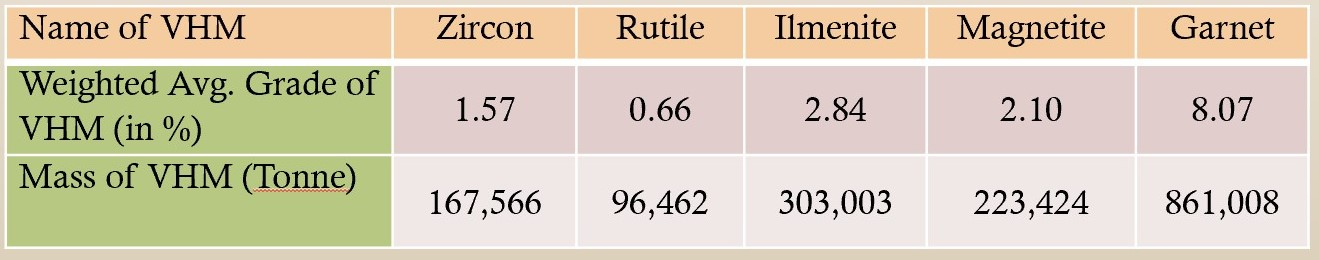• Resource for the proposed Mining Block-C up to a depth of 10 meters have been calculated using 8.51% weighted average of HM